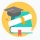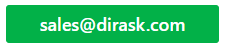Home
IT Knowledge
IT Career
Inspiration
Languages
EN

# Java - delete node from double linked list (custom implementation)

12 points
Created by:AnnLen
9180

## 1. Overview

In this post we will see how to implement algorithm to correctly delete node from double linked list. Below we have 2 implementations how to remove node:

• First one with node based on Integer
• Second one with generic Node and usage example based on String

When we unlink node from double linked list, we need to consider 4 cases:

• when list is empty (size is 0 and first and last node is null)
• when we need to delete first node (node that have null as previous node)
• when we need to delete middle node (node that has previous and next node)
• when we need to delete last node (node that have null as next node)

## 2. Delete node code example

Below we have java implementation with Node with Integer as item of double linked list with unlink method which will remove node from our list. This implementation is without generics to have this example as simple as possible. Generic implementation is in next part of this post.

``````package com.dirask;

Node first;
Node last;
int size;

Node tmpLast = last;
Node newNode = new Node(tmpLast, item, null);
last = newNode; // assign new node as last
if (tmpLast == null) {
first = newNode; // if list is empty
} else {
// take care that the last node will be linked
tmpLast.next = newNode;
}
size++;
return newNode;
}

if (prev == null) {
first = next;
} else {
prev.next = next;
}

if (next == null) {
last = prev;
} else {
next.prev = prev;
}

size--;
return item;
}

public int getSize() {
return size;
}

@Override
public String toString() {
if (first != null) {
return first.printForward();
}
return "List is empty";
}

private static class Node {
Node prev;
Integer item;
Node next;

public Node(Node prev, Integer item, Node next) {
this.prev = prev;
this.item = item;
this.next = next;
}

public String printForward() {
if (next != null) {
return item + " -> " + next.printForward();
}
return String.valueOf(item);
}
}

private static void print(DoubleLinkedList list) {
System.out.println("size: " + list.getSize() + " | list: " + list.toString());
}

public static void main(String[] args) {

print(list);

print(list);

print(list);

print(list);
}
}``````

Output:

``````size: 3 | list: 1 -> 2 -> 3
size: 2 | list: 2 -> 3
size: 1 | list: 3
size: 0 | list: List is empty``````

## 3. Delete node - generic implementation

Below we have java generic implementation of double linked list with unlink method which will remove node from our list.

``````package com.dirask;

private Node<E> first;
private Node<E> last;
private int size;

Node<E> tmpLast = last;
Node<E> newNode = new Node<>(tmpLast, item, null);
last = newNode; // assign new node as last
if (tmpLast == null) {
first = newNode; // if list is empty
} else {
// take care that the last node will be linked
tmpLast.next = newNode;
}
size++;
return newNode;
}

if (prev == null) {
first = next;
} else {
prev.next = next;
}

if (next == null) {
last = prev;
} else {
next.prev = prev;
}

size--;
return item;
}

public int getSize() {
return size;
}

@Override
public String toString() {
if (first != null) {
return first.printForward();
}
return "List is empty";
}

private static class Node<E> {
Node<E> prev;
E item;
Node<E> next;

public Node(Node<E> prev, E item, Node<E> next) {
this.prev = prev;
this.item = item;
this.next = next;
}

public String printForward() {
if (next != null) {
return item + " -> " + next.printForward();
}
return String.valueOf(item);
}
}

private static void print(DoubleLinkedList list) {
System.out.println("size: " + list.getSize() + " | list: " + list.toString());
}

public static void main(String[] args) {

print(list);

print(list);

print(list);

print(list);
}
}``````

Output:

``````size: 3 | list: 1 -> 2 -> 3
size: 2 | list: 2 -> 3
size: 1 | list: 3
size: 0 | list: List is empty``````

## References (Harvard CS classes - Data Structures)